## Place value in the millions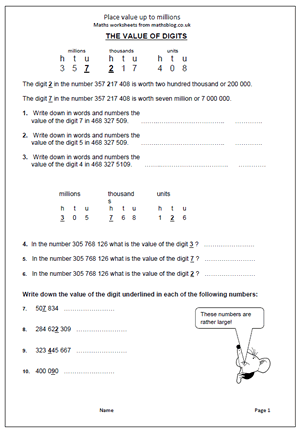Children are expected to work with even larger numbers in Year 5, including millions. Many will have difficulties in reading and writing such large numbers and will need a lot of help to appreciate the place value of each digit.

The easiest way to read  large numbers is to think of them as blocks of three, starting on the right.

Each block of three is hundreds, tens and units, which keep repeating themselves.

The first block of three are HUNDREDS, TENS AND UNITS of ones or units.

So  456 is four hundred and fifty six (units).

The second block of three are HUNDREDS, TENS AND UNITS of thousands.

So 456 456 is four hundred and fifty six thousand four hundred and fifty six.

This page takes a closer look at reading large numbers and finding the value of various digits.

Place value up to millions

## Counting in multiples of 6, 7, 8 and 9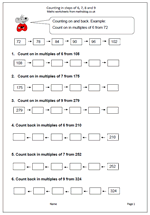A new target in the Maths Primary Framework for Year 4 is to count up in steps of 6, 7, and 9. This might sound easy and if the numbers are low there should not be a problem. However, counting up and down in steps of 6 or 7 starting from larger numbers can be tricky and might well involve a great deal of finger counting. Don’t be surprised at this as there is a lot of mathematical knowledge needed to successfully and quickly count up 7 from a number such as 196. It can be done by counting in single digits, or counting 4 to 200 and then 3, or even adding 10 and subtracting 3.

Counting in steps of 6,7,8 and 9

## Five times table in Year 2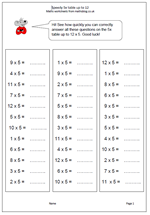Learning the five times table has now been placed firmly in the Year 2 Programme of Study and will prove quite a challenge for many children who find counting up to 100 hard, let alone reciting the 5 times table. To make it even more of a challenge the table now has to be learned up to 12 times 5 rather than just 10 times 5. There is a danger with this that children will learn the table ‘parrot fashion’ with little idea of what it means or how to use the knowledge.

There is, of course, a rhythm and pattern to the five times table, as alternate answers end in zero or 5. This makes it one of the easiest times tables to learn. My latest worksheet can be used as a timed practice, with three sets of 12 questions on the page.

Speedy 5x table

## New Maths Curriculum: Year 2 Statistics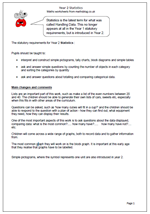Statistics is the latest term for what was called Handling Data. This no longer appears at all in the Year 1 statutory requirements, but is introduced in Year 2.

The statutory requirements for Year 2 Statistics :

Pupils should be taught to:

•    interpret and construct simple pictograms, tally charts, block diagrams and simple tables

•    ask and answer simple questions by counting the number of objects in each category and sorting the categories by quantity

Lists are an important part of this work, such as make a list of the even numbers between 20 and 40. The children should be able to generate their own lists of cars, sweets etc, especially when this fits in with other areas of the curriculum.

Questions can be asked, such as ‘how many cubes will fit in a cup?’ and the children should be able to respond to the question with a plan of action – how they can find out, what equipment they need, how they can display their results.

One of the most important aspects of this work is to ask questions about the data displayed, comparing data: what is the most common?….. how many have?…..    how many have not?…. etc.

Children will come across a wide range of graphs, both to record data and to gather information from.

The most common graph they will work on is the block graph. It is important at this early age that they realise that graphs have to be labelled.

Simple pictograms, where the symbol represents one unit are also introduced in year 2.

New Maths Curriculum: Statistics

## New Maths Curriculum: Year 2 Geometry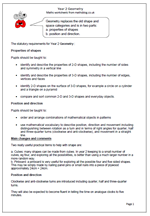Geometry replaces the old shape and space categories and is in two parts:

a. properties of shapes             b. position and direction.

The statutory requirements for Year 2 Geometry :

Properties of shapes

Pupils should be taught to:

•    identify and describe the properties of 2-D shapes, including the number of sides and symmetry in a vertical line

•    identify and describe the properties of 3-D shapes, including the number of edges, vertices and faces

•    identify 2-D shapes on the surface of 3-D shapes, for example a circle on a cylinder and a triangle on a pyramid

•    compare and sort common 2-D and 3-D shapes and everyday objects.

Position and direction

Pupils should be taught to:

•    order and arrange combinations of mathematical objects in patterns

•    use mathematical vocabulary to describe position, direction and movement including distinguishing between rotation as a turn and in terms of right angles for quarter, half and three-quarter turns (clockwise and anti-clockwise), and movement in a straight line.

Two really useful practical items to help with shape are:

a. Cubes: many shapes can be made from cubes. In year 2 keeping to a small number of cubes, eg four, and exploring all the possibilities, is better than using a much larger number in a more random way.
b. Pinboard: a pinboard is very useful for exploring all the possible four and five sided shapes. This may be simply made by nailing panel pins or small nails into a piece of plywood approximately 24cm × 24cm.

Position and direction
Clockwise and anti-clockwise turns are introduced including quarter, half and three-quarter turns.
They will also be expected to become fluent in telling the time on analogue clocks to five minutes.

New Maths Curriculum: Year 2 Geometry

## New Maths Curriculum: Year 2 Measurement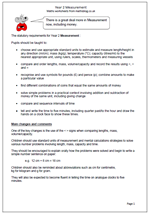The statutory requirements for Year 2 Measurement :

Pupils should be taught to:

•    choose and use appropriate standard units to estimate and measure length/height in any direction (m/cm); mass (kg/g); temperature (°C); capacity (litres/ml) to the nearest appropriate unit, using rulers, scales, thermometers and measuring vessels

•    compare and order lengths, mass, volume/capacity and record the results using >, < and =

•    recognise and use symbols for pounds (£) and pence (p); combine amounts to make a particular value

•    find different combinations of coins that equal the same amounts of money

•    solve simple problems in a practical context involving addition and subtraction of money of the same unit, including giving change

•    compare and sequence intervals of time

•    tell and write the time to five minutes, including quarter past/to the hour and draw the hands on a clock face to show these times.

One of the key changes is the use of the < > signs when comparing lengths, mass, volume/capacity.

Children should use standard units of measurement and mental calculations strategies to solve various number problems involving length, mass, capacity and time.

They should be encouraged to explain orally how the problems were solved and begin to write a simple number sentence on paper:

e.g.  12 cm + 6 cm = 18 cm

Children should also be reminded about abbreviations such as cm for centimetre,
kg for kilogram and g for gram.

They will also be expected to become fluent in telling the time on analogue clocks to five minutes.

New Maths Curriculum: Year 2 Measurement

## New Maths Curriculum: Year 2 Multiplication and Division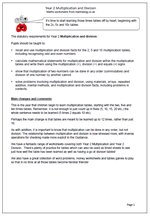Year 2 Multiplication and Division

It’s time to start learning those times tables off by heart; beginning with the 2x, 5x and 10x tables.
The statutory requirements for Year 2 Multiplication and division :

Pupils should be taught to:

• recall and use multiplication and division facts for the 2, 5 and 10 multiplication tables, including recognising odd and even numbers
• calculate mathematical statements for multiplication and division within the multiplication tables and write them using the multiplication (×), division (÷) and equals (=) signs
• show that multiplication of two numbers can be done in any order (commutative) and division of one number by another cannot
• solve problems involving multiplication and division, using materials, arrays, repeated addition, mental methods, and multiplication and division facts, including problems in contexts.

This is the year that children begin to learn multiplication tables, starting with the two, five and ten times tables. Remember, it is not enough to just count up in fives (5, 10, 15, 20 etc.) the whole sentence needs to be learned (5 times 2 equals 10 etc).
Perhaps the main change is that tables are meant to be learned up to 12 times, rather than just 10.

As with addition, it is important to know that multiplication can be done in any order, but not division. The relationship between multiplication and division is now stressed more, with inverse operations for checking made more explicit in the Guidance.

Year 2 multiplication and division statutory requirements

## New Maths Curriculum: Year 2 Addition and Subtraction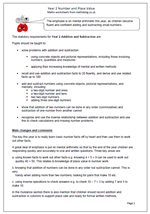Year 2 Addition and subtraction

The emphasis is on mental arithmetic this year, as children become fluent and confident adding and subtracting small numbers.

The statutory requirements for Year 2 Addition and Subtraction are:

Pupils should be taught to:

•    solve problems with addition and subtraction:

using concrete objects and pictorial representations, including those involving numbers, quantities and measures

applying their increasing knowledge of mental and written methods

•    recall and use addition and subtraction facts to 20 fluently, and derive and use related facts up to 100

•    add and subtract numbers using concrete objects, pictorial representations, and mentally, including:
a two-digit number and ones
a two-digit number and tens
two two-digit numbers

•    show that addition of two numbers can be done in any order (commutative) and subtraction of one number from another cannot

•    recognise and use the inverse relationship between addition and subtraction and use this to check calculations and missing number problems.

The key this year is to really learn basic number facts off by heart and then use them to work out other facts.
A great deal of emphasis is put on mental arithmetic so that by the end of the year children are responding quickly and accurately to oral and written questions. Three key areas are:

a. using known facts to work out other facts e.g. knowing 4 + 5 = 9 can be used to work out
quickly 40 + 50. This relates to knowledge of place value in number work.

b. knowing that addition of numbers can be done in any order but subtraction cannot. This is very
handy when adding more than two numbers; looking for pairs that make 10 etc.

c. using inverse operations to check answers e.g. to check 10 – 7 = 3 by adding 7 and 3 to make 10.

In the Guidance section there is also mention that children should record addition and subtraction in columns to support place vale and ready for formal written methods.

New Maths Programme of Study: Year 2 Addition and Subtraction

## New Maths Curriculum: Year 2 Number and Place Value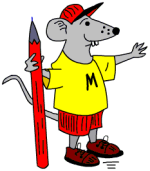There are some tough challenges in the new Year 2 Programme of Study. Let’s begin by looking at ‘Number and place value’.
The statutory requirements for Year 2 Number and Place Value are:

Number and place value

Pupils should be taught to:

•    count in steps of 2, 3, and 5 from 0, and in tens from any number, forward or backward

•    recognise the place value of each digit in a two-digit number (tens, ones)

•    identify, represent and estimate numbers using different representations, including the number line

•    compare and order numbers from 0 up to 100; use <, > and = signs

•    read and write numbers to at least 100 in numerals and in words

•    use place value and number facts to solve problems.

Main changes
Most of this looks very similar to the old curriculum. The main difference is the introduction of the < and > signs. Remembering which is which could prove very tricky.
Writing numbers to at least 100 in words is a real challenge which quite a few children will not be able to manage by the end of the year.
A key idea in this category is partitioning numbers in different ways. For example:
24 can be split up or partitioned into 20 + 4 or 10 + 14.
This is important to understand as it helps with written addition and subtraction in later years. Also it is important to begin to understand zero as a place holder; i.e. the 1 in 100 has a value ten times the 1 in 10, with the zeros being used as place holders.
Counting in multiples of 3 is a new target, introduced to help later with understanding the concept of a third.
Also note that this new programme will not become a statutory requirement until 2015.

Year 2 Number and Place Value

## New Maths Curriculum: Year 1 Measurement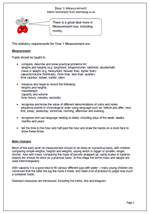Here are the statutory requirements for Measurement in Year 1. There is a great deal more in Measurement now, including money and time.

Measurement

Pupils should be taught to:

•    compare, describe and solve practical problems for:
lengths and heights (e.g. long/short, longer/shorter, tall/short, double/half)
mass or weight (e.g. heavy/light, heavier than, lighter than)
capacity/volume (full/empty, more than, less than, quarter)
time (quicker, slower, earlier, later)

•    measure and begin to record the following:
lengths and heights
mass/weight
capacity and volume
time (hours, minutes, seconds)

•    recognise and know the value of different denominations of coins and notes
sequence events in chronological order using language such as: before and after, next,
first, today, yesterday, tomorrow, morning, afternoon and evening

•    recognise and use language relating to dates, including days of the week, weeks, months and years

•    tell the time to the hour and half past the hour and draw the hands on a clock face to show these times.

Main changes

Much of the early work on measurement should to be done on a practical basis, with children comparing simple lengths, heights and weights, saying which is bigger or smaller, longer, shorter. Also with mass, comparing the mass of parcels wrapped up, using scales to balance objects etc should be done on a practical basis. At this stage the terms mass and weight are used interchangeably.

With capacity it is a good idea to fill various different jugs with water – many young children are convinced that the taller the jug the more it holds, and need a lot of practice to judge how much a container holds.

Standard measures are introduced, including the metre, litre and kilogram.

Year 1 Measurement statutory requirements